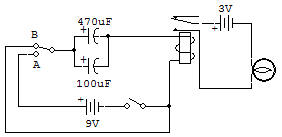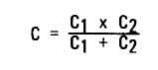Recherche personnalisé / personalised search

Momentary ON Call SwitchThe purpose of this experiment is to demonstrate a momentary ON circuit which is very easy to make. This type of circuit could be used where we would like to alert or call someone without causing an excessive amount of disturbance, such as in a hospital.

The circuit uses the capacitor storage theory discussed in project Capacitors Charge and Discharge in Capacitor Section. The difference is that here the energy is put to work energizing the Relay. In one position of the switch the Capacitors are charged by current which must flow through the Relay, and in the other position they are discharged by having the current flow through the Relay.

The capacitance used is high enough to keep the Relay energized for about a second. You may want to experiment with different amounts of capacitance.

Remember, when connecting Capacitors in parallel, the resultant capacitance equals the sum of the individual capacitances.

When connected in series capacitance must be calculated with the formula given below:Therefore if the 470 and 100 are rewired for a series connection, the resulting capacitance is aboutRecherche personnalisée